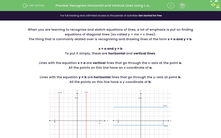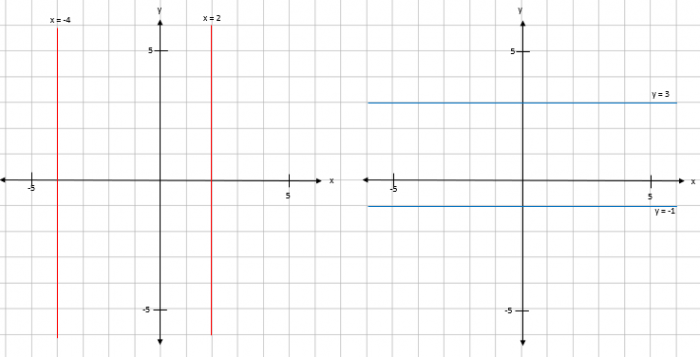# Recognise Horizontal and Vertical Lines Using x and y Coordinates

In this worksheet, students will learn how to recognise horizontal (y = b) and vertical (x = a) lines.Key stage:  KS 4

Year:  GCSE

GCSE Subjects:   Maths

GCSE Boards:   AQA, Eduqas, OCR, Pearson Edexcel,

Curriculum topic:   Algebra, Graphs of Equations and Functions

Curriculum subtopic:   Graphs Straight Line Graphs

Difficulty level:#### Worksheet Overview

When you are learning to recognise and sketch equations of lines, a lot of emphasis is put on finding equations of diagonal lines (so called y = mx + c lines).

The thing that is commonly skated over is recognising and drawing lines of the form x = a and y = b.

x = a and y = b

To put it simply, these are horizontal and vertical lines.

Lines with the equation x = a are vertical lines that go through the x-axis at the point a.

All the points on this line have an x coordinate of a.

Lines with the equation y = b are horizontal lines that go through the y-axis at point b.

All the points on this line have a y coordinate of b.Let's have a go at some questions.

### What is EdPlace?

We're your National Curriculum aligned online education content provider helping each child succeed in English, maths and science from year 1 to GCSE. With an EdPlace account you’ll be able to track and measure progress, helping each child achieve their best. We build confidence and attainment by personalising each child’s learning at a level that suits them.

Get started